# Introduction to DC-DC Converters

## 2/18/2016 | By Maker.io Staff

DC-DC converters are widely used to efficiently produce a regulated voltage from a source that may or may not be well controlled to a load that may or may not be constant. This paper briefly introduces DC-DC converters, notes common examples, and discusses important datasheet parameters and applications of DC-DC converters.

DC-DC converters are high-frequency power conversion circuits that use high-frequency switching and inductors, transformers, and capacitors to smooth out switching noise into regulated DC voltages. Closed feedback loops maintain constant voltage output even when changing input voltages and output currents. At 90% efficiency, they are generally much more efficient and smaller than linear regulators. Their disadvantages are noise and complexity.

DC-DC converters come in non-isolated and isolated varieties. Isolation is determined by whether or not the input ground is connected to the output ground.

Four common topologies that makers might find useful are the buck, boost, buck-boost, and SEPIC converters.

A buck converter steps a voltage down, producing a voltage lower than the input voltage. A buck converter could be used to charge a lithium ion battery to 4.2 V, from a 5 V USB source.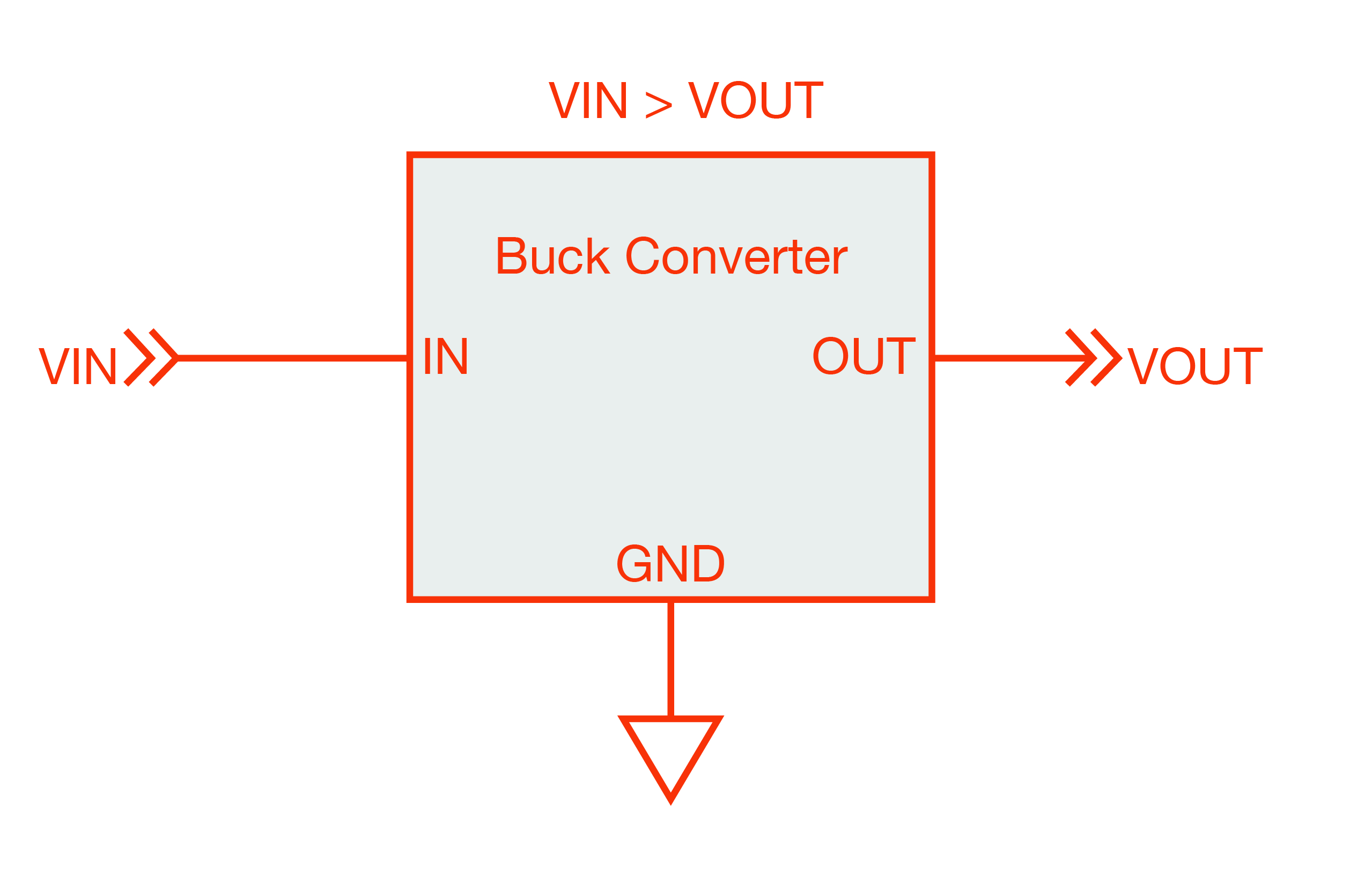Figure 1: Buck converter

A boost converter steps a voltage up, producing a voltage higher than the input voltage. A boost converter could be used to drive a string of LEDs from a lithium cell, or provide a 5 V USB output from a lithium cell.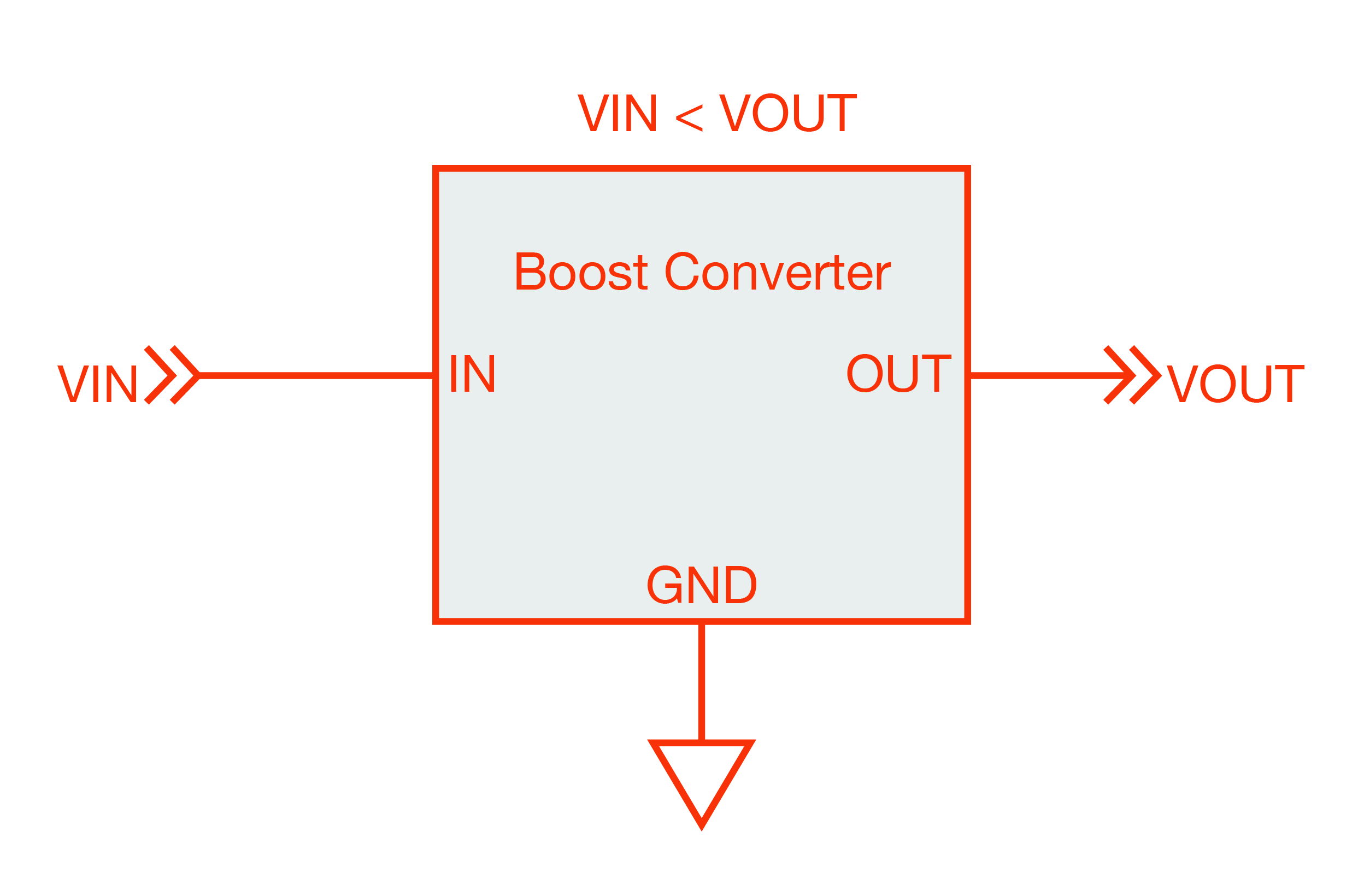Figure 2: Boost converter

A buck-boost converter steps a voltage up or down, producing a voltage equal to or higher or lower than the input voltage. A buck boost could be used to provide a 12 V output from a 12 V battery. A 12V battery’s voltage can vary between 10 V and 14.7 V. A buck boost could also power an LED from a single cell. An LED forward drop is as high as 3 V. A lithium battery cell can vary between 2.5 and 4.2 V. There are buck-boosts that produce positive and negative voltages.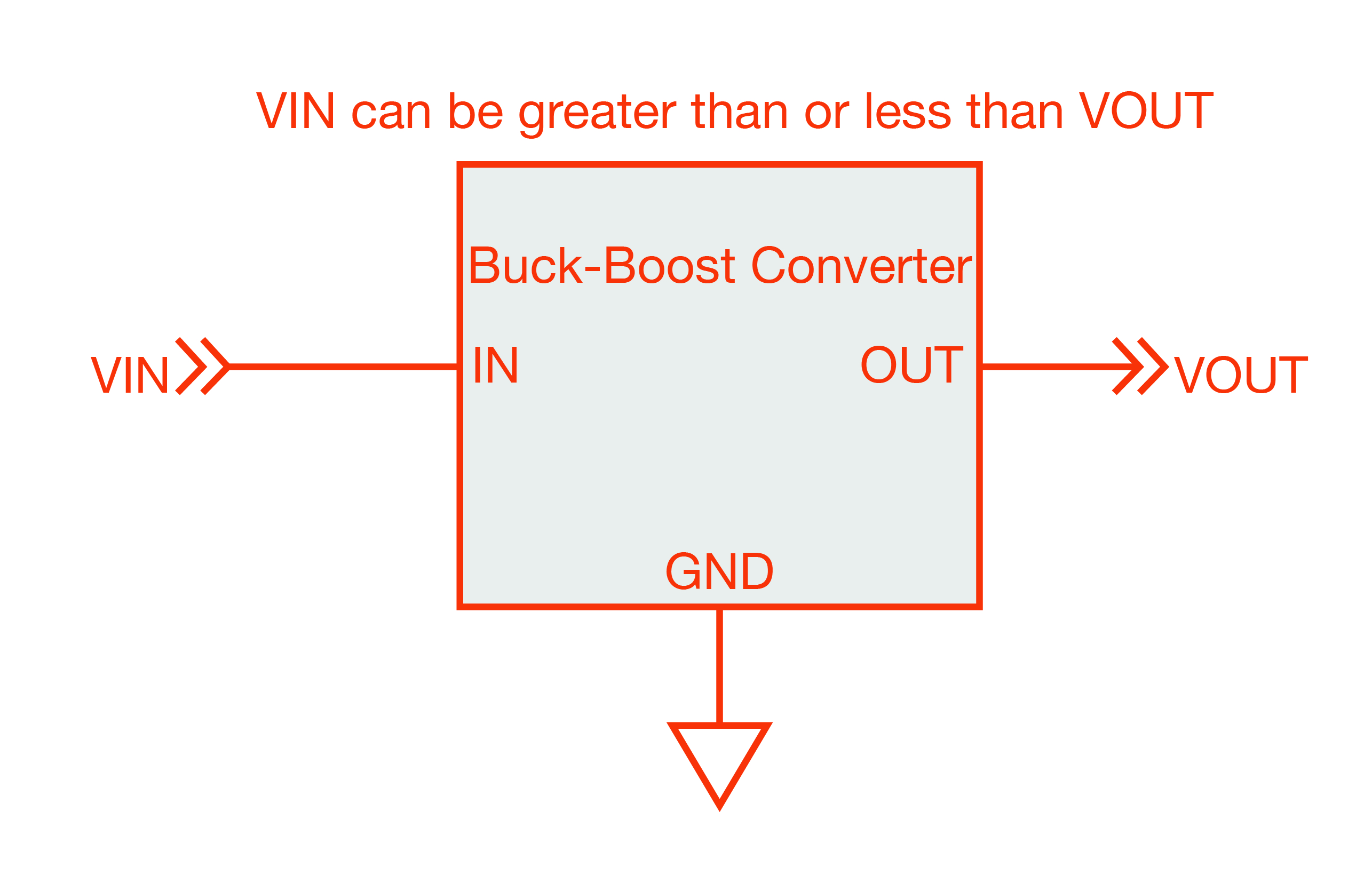Figure 3: Buck-boost converter

A SEPIC converter also steps a voltage up or down, producing a voltage equal to or higher or lower than the input voltage. A SEPIC is used for similar applications as the buck-boost, but provides some advantages in some applications.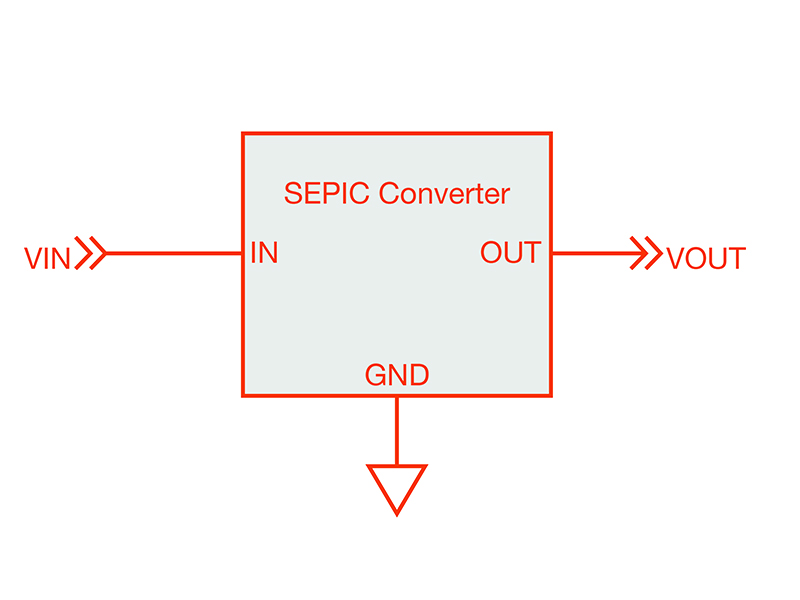Figure 4: SEPIC converter

Important examples of isolated DC-DC converters are the forward and flyback converter. The forward converter is an isolated buck converter. The flyback is an isolated boost converter. Isolated power converters are those in which the input ground and output ground are not connected. This can be used to produce positive and negative voltages depending on how you connect the output terminals. See figure 5 for examples of creating positive and negative output voltages with isolated DC-DC converters.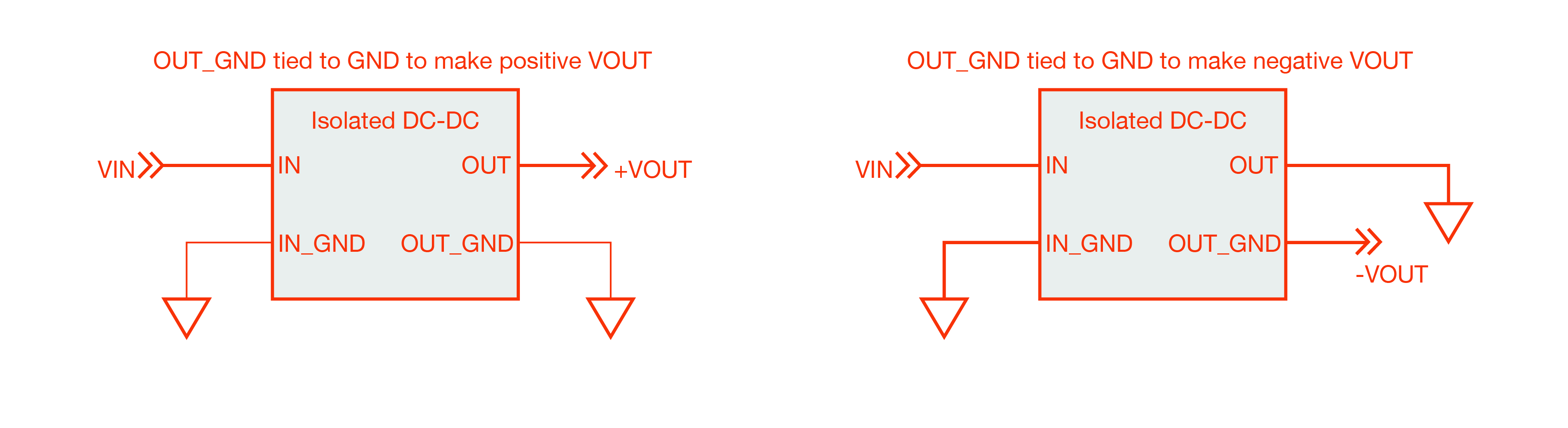Figure 5: Using isolated DC-DC converters to create positive and negative output voltages

Important performance characteristics

DC-DC converter datasheets have key parameters that describe their important characteristics. The following are some important characteristics to consider in designs.

Efficiency

Efficiency is the fraction of input power that reaches the load.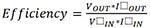Some DC-DC converters are 90%+ efficient. When using a DC-DC converter, it is good practice to make sure that the source providing power to the DC-DC converter can provide enough power to account for the inefficiency. A good rule of thumb is assume a DC-DC converter is 80% efficient, and then use a source with 125% the load power. For example, if a 4 W load is needed, use a 4 W DC-DC, driven by a 5W source. Efficiency for DC-DC converters is typically specified in curves, with peak efficiency achieved at certain load current. Efficiency may be lower at lower power outputs, where the amount of power required to power the circuit is comparable to the load power.

Current rating

This is the maximum amount of current that a user should try to have the DC-DC converter provide to a load. The DC-DC converter may provide more than this amount of current, but will get hot, and may fail.

Temperature rating

This is the maximum ambient temperature that the DC-DC converter should run in under full load. If pushed past this safety limit, the DC-DC converter may overheat and be damaged, or may shut down as a protective measure.

Ripple voltage

This rating is a measure of how much ripple voltage is on the output. Be sure that a buck converter’s voltage ripple rating meets your needs.

Regulation

This figure of merit is related to how tightly the output is controlled over input voltage and load current. If a DC-DC converter has a regulation rating of 1%, then the output voltage will not deviate more than 1% from the nominal value over the specified input voltage range and output current range.

Transient response

DC-DC converters use closed feedback loops to provide a regulated output. Changes in load current or input voltage can cause temporary shifts. Speed of control loop response gives you an idea of how long it takes for the buck converter to respond to changing conditions and get the output voltage in regulation.

Voltage rating

DC-DC converters have limits on how far up or how far down they can transform a voltage.

Size and weight

DC-DC converters can operated at very high frequencies, enabling them to be made small. Because some loss mechanisms increase with frequency, causing them to be less efficient, there is somewhat of a trade-off between size and efficiency.

Conclusion

This paper has discussed DC-DC converters, introduced important isolated and non-isolated topologies, and covered some important datasheet parameters.